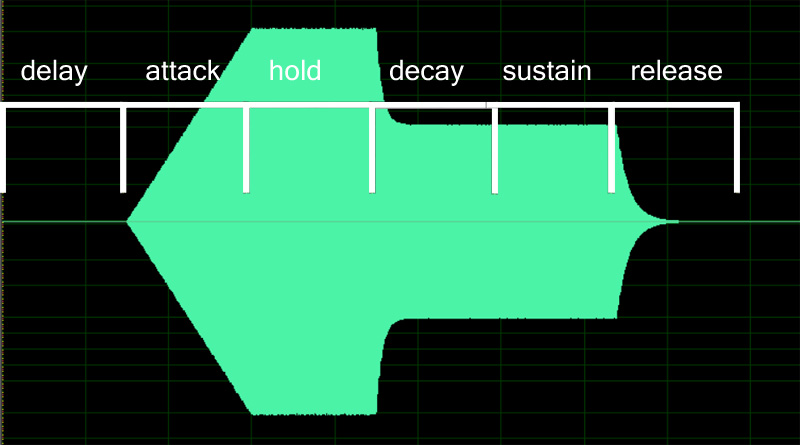ampeg_attack

EG attack time.

ampeg_attack=1.2
fileg_attack=0.1

Practical Considerations #

These are very frequently used, especially with amplifier envelopes. ampeg_attack is the standard “A” in the basic ADSR volume envelope. fileg_attack is key to 303-style basses.

In ARIA, the SFZ1 envelopes have linear attack (for pitcheg and fileg, probably linear in cents, which won’t translate into linear in Hertz). Decay and release stages have a curve which is faster than linear, and it seems to match “well enough” with a multiplicatively decreasing curve. The step size should be close to $$\mu = \exp \left( - \frac{8.0}{t \times s} \right)$$ where $$t$$ is the decay duration in seconds, and $$s$$ is the sample rate in Hertz. The envelope $$x_{n+1}$$ at index $$n+1$$ is thus computed as $$x_{n+1} = \mu \times x_{n}.$$

Here is a screenshot of a file output using Sforzando, showing the ampeg_envelope shape and its stages.Name Version Type Default Range Unit
ampeg_attack SFZ v1 float 0 0 to 100 seconds
Modulations
ampeg_attackccN float 0 -100 to 100 seconds
ampeg_vel2attack float 0 -100 to 100 seconds

Category: Modulation, Envelope Generators